Documentation

In Control System Toolbox™, PID Tuner provides system response plots and other tools for tuning PID controllers for plants represented by LTI models.

For information about analysis in PID Tuner with Simulink® models, see Analyze Design in PID Tuner (Simulink Control Design).

### Plot System Responses

To determine whether the compensator design meets your requirements, you can analyze the system response using the response plots. On the PID Tuner tab, select a response plot from the Add Plot menu. The Add Plot menu also lets you choose from several step plots (time-domain response) or Bode plots (frequency-domain response).For 1-DOF PID controller types such as PI, PIDF, and PDF, the software computes system responses based upon the following single-loop control architecture, where G is your specified plant and C is the PID controller:For 2-DOF PID controller types such as PI2, PIDF2, and I-PD, the software computes responses based upon the following architecture: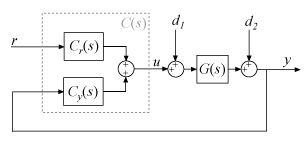The system responses are based on the decomposition of the 2-DOF PID controller, C, into a setpoint component Cr and a feedback component Cy, as described in Two-Degree-of-Freedom PID Controllers.

The following table summarizes the available responses for analysis plots. (For frequency-response-data plants such as `frd` models, time-domain response plots are not available.)

ResponsePlotted System (1-DOF)Plotted System (2-DOF)Description
`Plant`GGPlant response. Use to examine plant dynamics.
`Open-loop`GC–GCyResponse of the open-loop controller-plant system. Use for frequency-domain design.
Use when your design specifications include robustness criteria such as open-loop gain margin and phase margin.
`Reference tracking`

$\frac{GC}{1+GC}$ (from r to y)

$\frac{G{C}_{r}}{1-G{C}_{y}}$ (from r to y)

Closed-loop system response to a step change in setpoint. Use when your design specifications include setpoint tracking.
`Controller effort`

$\frac{C}{1+GC}$ (from r to u)

$\frac{{C}_{r}}{1-G{C}_{y}}$ (from r to u)

Closed-loop controller output response to a step change in setpoint. Use when your design is limited by practical constraints, such as controller saturation.
`Input disturbance rejection`

$\frac{G}{1+GC}$ (from d1 to y)

$\frac{G}{1-G{C}_{y}}$ (from d1 to y)

Closed-loop system response to load disturbance (a step disturbance at the plant input). Use when your design specifications include input disturbance rejection.
`Output disturbance rejection`

$\frac{1}{1+GC}$ (from d2 to y)

$\frac{1}{1-G{C}_{y}}$ (from d2 to y)

Closed-loop system response to a step disturbance at plant output. Use when you want to analyze sensitivity to modeling errors.

#### Compare Tuned Response to Baseline Response

If you have defined a baseline controller, then by default PID Tuner displays both the responses using the current PID Tuner design and the responses using the baseline controller.There are two ways to define a baseline controller:

• Load a baseline controller when you open PID Tuner, using the syntax `pidTuner(sys,C0)`.

• Make the current PID Tuner design the baseline controller at any time, by clicking the Export arrow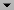and selecting ```Save as Baseline```.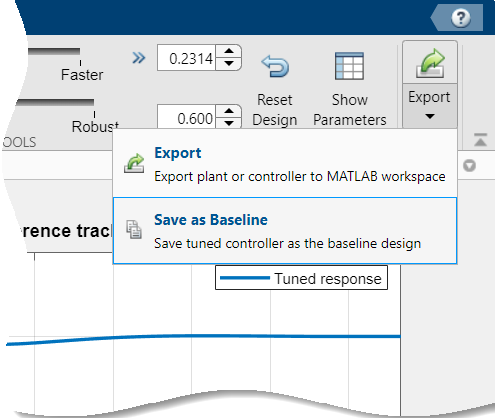When you do so, the current Tuned response becomes the Baseline response. Further adjustment of the current design creates a new Tuned response line.

To hide the Baseline response, clickOptions, and uncheck Show Baseline Controller Data.

### View Numeric Values of System Characteristics

You can view the values for system characteristics, such as peak response and gain margin, either:

• Directly on the response plot — Use the right-click menu to add characteristics, which appear as blue markers. Then, left-click the marker to display the corresponding data panel.

• In the Performance and robustness table — To display this table, click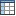Show Parameters.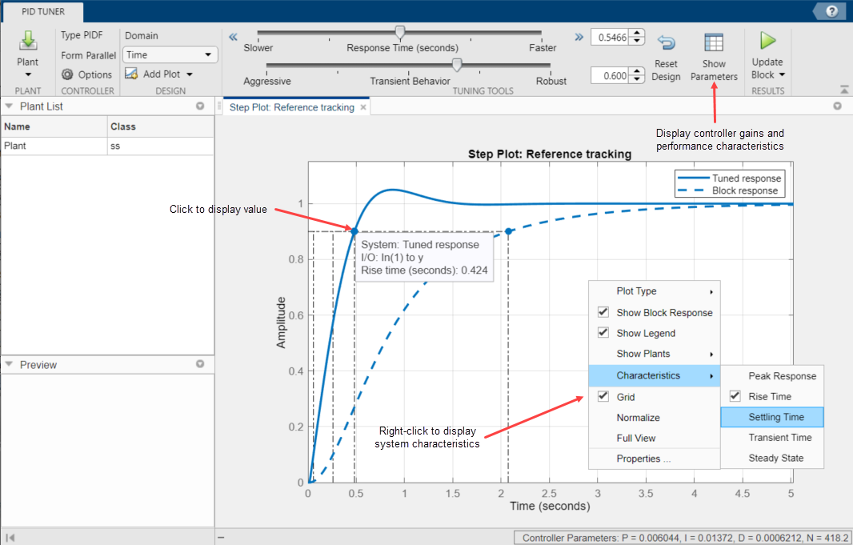### Refine the Design

If the response of the initial controller design does not meet your requirements, you can interactively adjust the design. PID Tuner gives you two Domain options for refining the controller design:

• `Time` domain (default) — Use the Response Time slider to make the closed-loop response of the control system faster or slower. Use the Transient Behavior slider to make the controller more aggressive at disturbance rejection or more robust against plant uncertainty.

• `Frequency` — Use the Bandwidth slider to make the closed-loop response of the control system faster or slower (the response time is 2/wc, where wc is the bandwidth). Use the Phase Margin slider to make the controller more aggressive at disturbance rejection or more robust against plant uncertainty.

In both modes, there is a trade-off between reference tracking and disturbance rejection performance. For an example that shows how to use the sliders to adjust this trade-off, see Tune PID Controller to Favor Reference Tracking or Disturbance Rejection (PID Tuner).

### Tip

To revert to the initial controller design after moving the sliders, click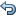Reset Design.